Reach Us+44-7480-724769
The Generalized Burnside Theorem in Noncommutative Deformation Theory | OMICS International
Journal of Generalized Lie Theory and Applications
All submissions of the EM system will be redirected to Online Manuscript Submission System. Authors are requested to submit articles directly to Online Manuscript Submission System of respective journal.

The Generalized Burnside Theorem in Noncommutative Deformation Theory

The Generalized Burnside Theorem in Noncommutative Deformation Theory*

Eivind Eriksen

BI Norwegian Business School, Department of Economics, N-0442 Oslo, Norway

*This article is a part of a Special Issue on Deformation Theory and Applications (A. Makhlouf, E. Paal and A. Stolin, Eds.).

Corresponding Author:
Eivind Eriksen
E-mail: [email protected]

Received Date: October 01, 2009; Accepted Date: January 26, 2011

Visit for more related articles at Journal of Generalized Lie Theory and Applications

Abstract

Let A be an associative algebra over a field k, and letMbe a finite family of right A-modules. A study of the noncommutative deformation functor DefM of the familyMleads to the construction of the algebra OA(M) of observables and the generalized Burnside theorem, due to Laudal (2002). In this paper, we give an overview of aspects of noncommutative deformations closely connected to the generalized Burnside theorem.

Introduction

Let k be a field and let A be an associative k-algebra. For any right A-moduleM, there is a commutative deformation functor Def M : l → Sets defined on the category l of local Artinian commutative k-algebras with residue field k. We recall that for an algebra R in l, a deformation of M to R is a pair (MR, τ), where MR is an R-A bimodule (on which k acts centrally) that is R-flat, and τ : k ⊕R MR → M is an isomorphism of right A-modules.

Let ar be the category of r-pointed Artinian k-algebras for r ≥ 1, the natural noncommutative generalization of l. We recall that an algebra R in ar is an Artinian ring, together with a pair of structural ring homomorphisms f : kr → R and g : R → kr with gof = id, such that the radical I(R) = ker(g) is nilpotent. Any algebra R in ar has r simple right modules of dimension one, the natural projections {k1, . . . , kr} of kr.

In , a noncommutative deformation functor DefM : ar → Sets of a finite family M = {M1, . . . , Mr} of right A-modules was introduced, as a generalization of the commutative deformation functor DefM : l → Sets of a right A-module M. In the case r = 1, this generalization is completely natural, and can be defined word for word as in the commutative case. The generalization to the caser > 1 is less obvious and has further-reaching consequences, but is still very natural. A deformation of M to R is defined to be a pair (MR, {τi}1≤i≤r), where MR is an R-A bimodule (on which k acts centrally) that is R-flat, and τi : kiRMR → Mi is an isomorphism of right A-modules for 1 ≤ i ≤ r. We remark that MR is R-flat if and only if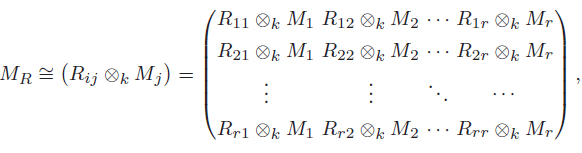considered as a left R-module, and that a deformation in DefM(R) may be thought of as a right multiplication A → EndR(MR) of A on the left R-module MR that lifts the multiplication ρ : A → ⊕i Endk(Mi) of A on the familyM.

There is an obstruction theory for DefM, generalizing the obstruction theory for the commutative deformation functor. Hence there exists a formal moduli (H,MH) for DefM (assuming a mild condition on M). We consider the algebra of observables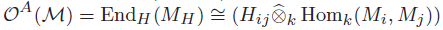and the commutative diagram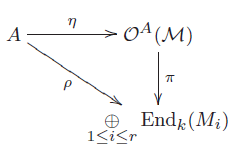given by the versal family MH ∈ DefM(H). The algebra B = OA(M) has an induced right action on the family Mextending the action of A, and we may considerMas a family of right B-modules. In fact,Mis the family of simple B-modules since π can be identified with the quotient morphism B → B/radB.

When A is an algebra of finite dimension over an algebraically closed field k and M is the family of simple right A-modules, Laudal proved the generalized Burnside theorem in , generalizing the structure theorem for semi-simple algebras and the classical Burnside theorem. Laudal’s result is stated in the following form.

Theorem (The generalized Burnside theorem). Let A be a finite-dimensional algebra over a field k, and letM = {M1,M2, . . . , Mr} be the family of simple right A-modules. If EndA(Mi) = k for 1 ≤ i ≤ r, then η : A → OA(M) is an isomorphism. In particular, η is an isomorphism when k is algebraically closed.

Let A be an algebra of finite dimension over an algebraically closed field k and letMbe any finite family of right A-modules of finite dimension over k. Then the algebra B = OA(M) has the property that ηB : B → OB(M) is an isomorphism, or equivalently, that the assignment (A,M) → (B,M) is a closure operation. This means that the family Mhas exactly the same module-theoretic properties, in terms of (higher) extensions and Massey products, considered as a family of modules over B as over A.

Noncommutative deformations of modules

Let k be a field. For any integer r ≥ 1, we consider the category ar of r-pointed Artinian k-algebras. We recall that an object in ar is an Artinian ring R, together with a pair of structural ring homomorphisms f : kr → R and g : R → kr with gof = id, such that the radical I(R) = ker(g) is nilpotent. The morphisms of ar are the ring homomorphisms that commute with the structural morphisms. It follows from this definition that I(R) is the Jacobson radical of R, and therefore that the simple right R-modules are the projections {k1, . . . , kr} of kr.

Let A be an associative k-algebra. For any familyM = {M1, . . . , Mr} of right A-modules, there is a noncommutative deformation functor DefM : ar → Sets, introduced by Laudal ; see also Eriksen . For an algebra R in ar, we recall that a deformation ofMover R is a pair (MR, {τi}1≤i≤r), where MR is an R-A bimodule (on which k acts centrally) that is R-flat, and τi : kiRMR → Mi is an isomorphism of right A-modules for 1 ≤ i ≤ r. Moreover,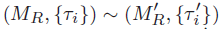are equivalent deformations over R if there is an isomorphism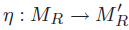of R-A bimodules such that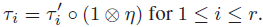We may prove that MR is R-flat if and only if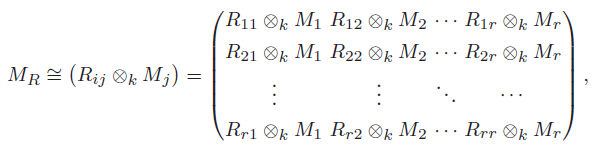considered as a left R-module, and a deformation in DefM(R) may be thought of as a right multiplication A → EndR(MR) of A on the left R-moduleMR that lifts the multiplication ρ : A→⊕i Endk(Mi) of A on the familyM.

Let us assume that M is a swarm, that is, Ext1A(Mi,Mj) has finite dimension over k for 1 ≤ i, j ≤ r. Then DefM has a pro-representing hull or a formal moduli (H,MH); see Laudal [2, Theorem 3.1]. This means that H is a complete r-pointed k-algebra in the pro-category âr, and that MH ∈ DefM(H) is a family defined over H with the following versal property: for any algebra R in ar and any deformation MR ∈ DefM(R), there is a homomorphism φ : H → R such that DefM(φ)(MH) = MR. The formal moduli (H,MH) is unique up to non-canonical isomorphism. However, the morphism φ is not uniquely determined by (R,MR).

WhenMis a swarm with formal moduli (H,MH), right multiplication on the H-A bimodule MH by elements in A determines an algebra homomorphism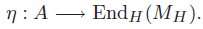We write OA(M) = EndH(MH) and call it the algebra of observables. Since MH is H-flat, we have that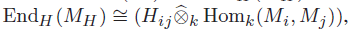and it follows that OA(M) is explicitly given as the matrix algebra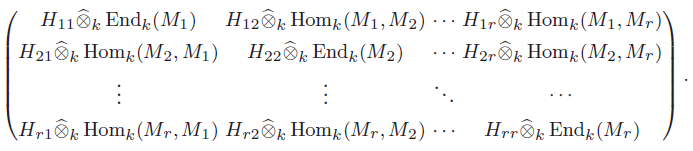Let us write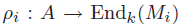for the structural algebra homomorphism defining the right A-module structure on Mi for 1 ≤ i ≤ r, and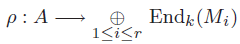for their direct sum. Since H is a complete r-pointed algebra in âr, there is a natural morphism H → kr, inducing an algebra homomorphism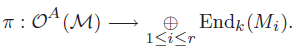By construction, there is a right action of OA(M) on the familyMextending the right action of A, in the sense that the diagram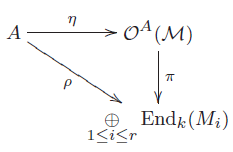commutes. This makes it reasonable to call OA(M) the algebra of observables.

The generalized Burnside theorem

Let k be a field and let A be a finite-dimensional associative k-algebra. Then the simple right modules over A are the simple right modules over the semi-simple quotient algebra A/ rad(A), where rad(A) is the Jacobson radical of A. By the classification theory for semi-simple algebras, it follows that there are finitely many non-isomorphic simple right A-modules.

We consider the noncommutative deformation functor DefM : ar → Sets of the family M = {M1,M2, . . . , Mr} of simple right A-modules. Clearly, M is a swarm, hence DefM has a formal moduli (H,MH), and we consider the commutative diagram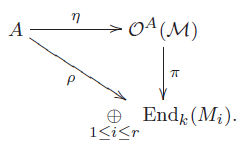By a classical result, due to Burnside, the algebra homomorphism ρ is surjective when k is algebraically closed. This result is conveniently stated in the following form.

Theorem 1 (Burnside’s theorem).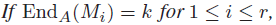then ρ is surjective. In particular, ρ is surjective when k is algebraically closed.

Proof. There is a factorization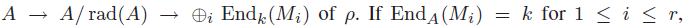then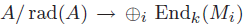is an isomorphism by the classification theory for semi-simple algebras. Since EndA(Mi) is a division ring of finite dimension over k, it is clear that EndA(Mi) = k whenever k is algebraically closed.

Let us write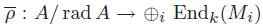for the algebra homomorphism induced by ρ. We observe that ρ is surjective if and only if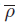is an isomorphism. Moreover, let us write J = rad(OA(M)) for the Jacobson radical of OA(M). Then we see that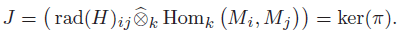Since ρ(radA) = 0 by definition, it follows that η(radA) ⊆ J. Hence there are induced morphisms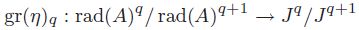for all q ≥ 0. We may identify gr(η)0 with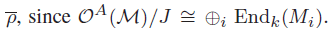The conclusion in Burnside’s theorem is therefore equivalent to the statement that gr(η)0 is an isomorphism.

Theorem 2 (The generalized Burnside theorem). Let A be a finite-dimensional algebra over a field k, and letM= {M1,M2, . . . , Mr} be the family of simple right A-modules. If EndA(Mi) = k for 1 ≤ i ≤ r, then η : A→OA(M) is an isomorphism. In particular, η is an isomorphism when k is algebraically closed.

Proof. It is enough to prove that η is injective and that gr(η)q is an isomorphism for q = 0 and q = 1, since A and OA(M) are complete in the rad(A)-adic and J-adic topologies. By Burnside’s theorem, we know that gr(η)0 is an isomorphism. To prove that η is injective, let us consider the kernel ker(η) ⊆ A. It is determined by the obstruction calculus of DefM; see Laudal [2, Theorem 3.2] for details. When A is finite-dimensional, the right regular A-module AA has a decomposition series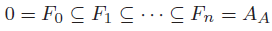with Fp/Fp−1 a simple right A-module for 1 ≤ p ≤ n. Namely, AA is an iterated extension of the modules inM. This implies that η is injective; see Laudal [2, Corollary 3.1]. Finally, we must prove that gr(η)1 : rad(A)/ rad(A)2 → J/J2 is an isomorphism. This follows from the Wedderburn-Malcev theorem; see Laudal [2, Theorem 3.4], for details.

Properties of the algebra of observables

Let A be a finite-dimensional algebra over a field k, and letM= {M1, . . . , Mr} be any family of right A-modules of finite dimension over k. Then M is a swarm, and we denote the algebra of observables by B = OA(M). It is clear that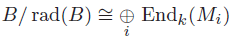is semi-simple, and it follows that M is the family of simple right B-modules. In fact, we may show that M is a swarm of B-modules, since B is complete and B/(radB)n has finite dimension over k for all positive integers n.

Proposition 3. If k is an algebraically closed field, then ηB : B →OB(M) is an algebra isomorphism.

Proof. SinceMis a swarm of A-modules and of B-modules, we may consider the commutative diagram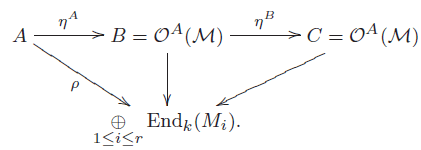The algebra homomorphism ηB induces maps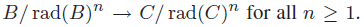Since k is algebraically closed and B/rad(B)n has finite dimension over k, it follows from the generalized Burnside theorem that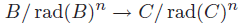is an isomorphism for all n ≥ 1. Hence ηB is an isomorphism.

In particular, the proposition implies that the assignment (A,M) → (B,M) is a closure operation when k is algebraically closed. In other words, the algebra B = OA(M) has the following properties:

(1) the familyMis the family of the simple B-modules;

(2) the family M has exactly the same module-theoretic properties, in terms of (higher) extensions and Massey products, considered as a family of modules over B as over A.

Moreover, these properties characterize the algebra B = OA(M) of observables.

Examples: representations of ordered sets

Let k be an algebraically closed field, and let Λ be a finite ordered set. Then the algebra A = k[Λ] is an associative algebra of finite dimension over k. The category of right A-modules is equivalent to the category of presheaves of vector spaces on Λ, and the simple A-modules correspond to the presheaves {Mλ : λ ∈ Λ} defined by Mλ(λ) = k and Mλ(λ’) = 0 forλ’ = λ. The following results are well known:A hereditary example

Let us first consider the following ordered set. We label the elements by natural numbers, and write i → j when i > j: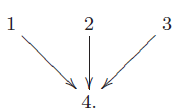In this case, the simple modules are given by M = {M1,M2,M3,M4}, and we can easily compute the algebra OA(M) of observables since Ext2A(Mi,Mj) = 0 for all 1 ≤ i, j ≤ 4. We obtain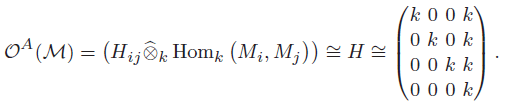It follows from the generalized Burnside theorem that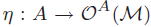is an isomorphism. Hence we recover the algebra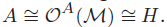The diamond

Let us also consider the following ordered set, called the diamond. We label the elements by natural numbers, and write i → j when i > j: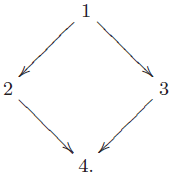In this case, the simple modules are given by M = {M1,M2,M3,M4}. Since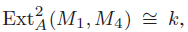we must compute the cup-products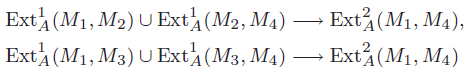in order to compute H. These cup-products are non-trivial; see Laudal [2, Remark 3.2] for details. Hence we obtain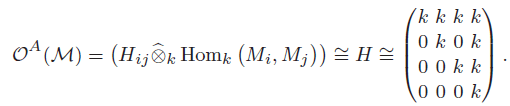Note that H14 is two-dimensional at the tangent level and has a relation. Also in this case, it follows from the generalized Burnside theorem that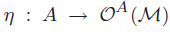is an isomorphism. Hence we recover the algebra A ≅ OA(M) ≅ H.

References

Select your language of interest to view the total content in your interested language